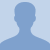# Binary Boiling Point Diagram of a Liquid-Liquid MixtureThis lesson will look at a Binary Boiling Point Diagram of a Liquid-Liquid Mixture. This diagram will show temperature, mole fraction of toluene and the mole fraction of benzene. This lesson will also look at a... This lesson will look at a Binary Boiling Point Diagram of a Liquid-Liquid Mixture. This diagram will show temperature, mole fraction of toluene and the mole fraction of benzene. This lesson will also look at a digram to explain how to solve for the amount of molecules.
More... Collapse
59 Views